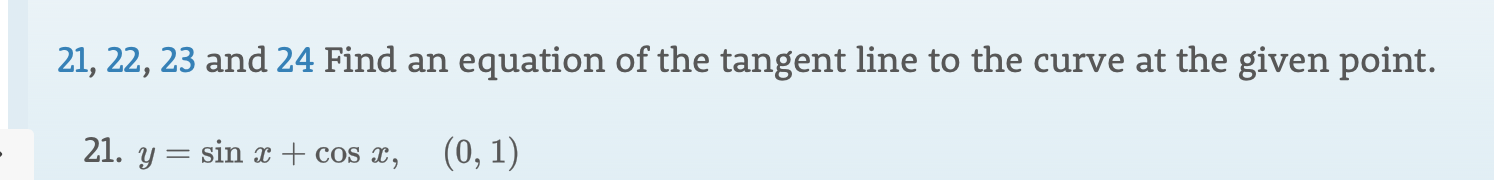# 21, 22, 23 and 24 Find an equation of the tangent line to the curve at the given point.21. y= sin x + cos x(0, 1)

Question
10 views

please answer the question provided in the image and show all work. thank youhelp_outlineImage Transcriptionclose21, 22, 23 and 24 Find an equation of the tangent line to the curve at the given point. 21. y= sin x + cos x (0, 1) fullscreen
check_circle

star
star
star
star
star
1 Rating
Step 1

The given function is,

Step 2

Differentiate f(x) with respect to x.

Step 3

In order to find the slope of the line su...

### Want to see the full answer?

See Solution

#### Want to see this answer and more?

Solutions are written by subject experts who are available 24/7. Questions are typically answered within 1 hour.*

See Solution
*Response times may vary by subject and question.
Tagged in

### Derivative